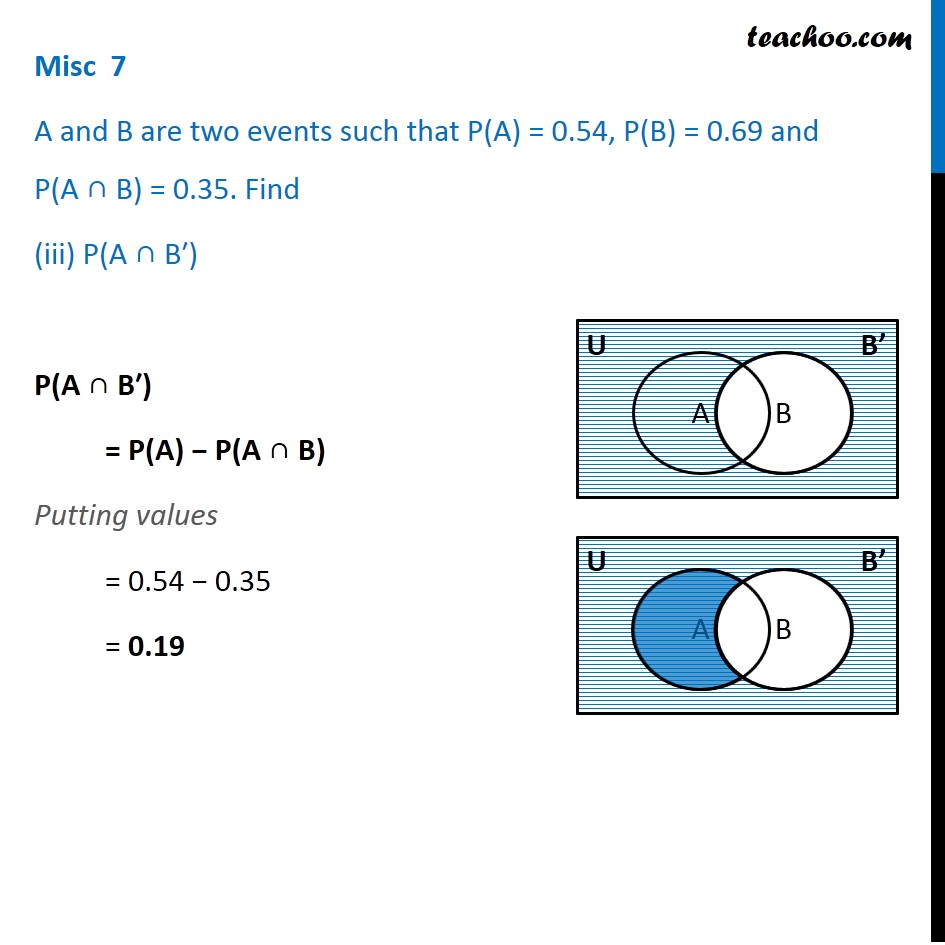Miscellaneous

Chapter 14 Class 11 Probability
Serial order wiseLearn in your speed, with individual attention - Teachoo Maths 1-on-1 Class

### Transcript

Misc 7 A and B are two events such that P(A) = 0.54, P(B) = 0.69 and P(A ∩ B) = 0.35. Find (iii) P(A ∩ B′) P(A ∩ B′) = P(A) − P(A ∩ B) Putting values = 0.54 − 0.35 = 0.19